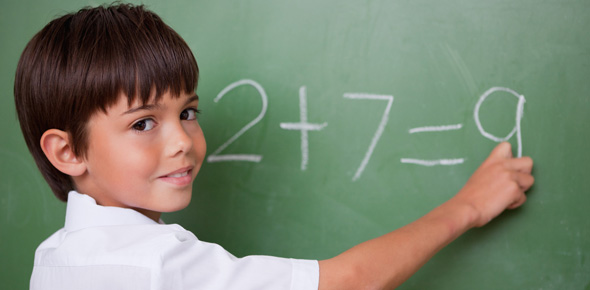# Ultimate Quiz On Simple Addition!

10 QuestionsSettingsThis quiz is meant for age group 9 to 13 yrs old. Please look at the problems and answer them using the multiple choice. Just test how much time you will take to add the numbers. This is basic addition. So let's try out this quiz. All the best!

Related Topics
• 1.
3+15=
• A.

18

• B.

12

• C.

9

• D.

4

• E.

10

• 2.
12+5=
• A.

9

• B.

11

• C.

5

• D.

18

• E.

17

• 3.
6+4=
• A.

2

• B.

14

• C.

8

• D.

10

• E.

16

• 4.
17+3=
• A.

0

• B.

20

• C.

10

• D.

4

• E.

7

• 5.
25+36
• A.

64

• B.

61

• C.

62

• D.

63

• 6.
99+22=
• A.

121

• B.

125

• C.

142

• D.

136

• 7.
105+85=
• A.

188

• B.

190

• C.

196

• D.

186

• 8.
45+23
• A.

68

• B.

69

• C.

88

• D.

75

• 9.
21+62
• A.

80

• B.

83

• C.

84

• D.

86

• 10.
25+88
• A.

113

• B.

112

• C.

116

• D.

119